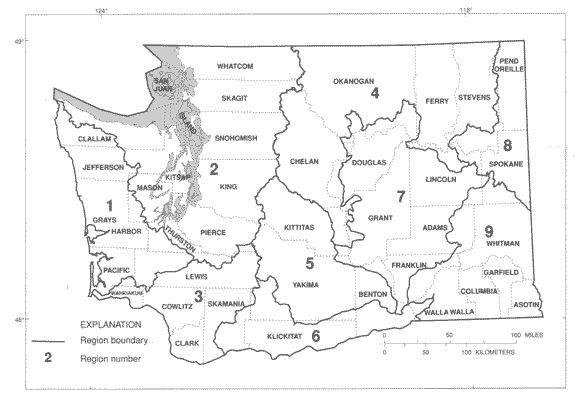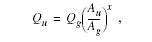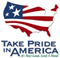# The National Flood-Frequency Program—Methods for Estimating Flood Magnitude and Frequency in Washington, 2001

#### U.S. Geological Survey Fact Sheet 016-01 March, 2001

This report is available in pdf 111KB.

## Introduction

Estimates of the magnitude and frequency of flood-peak discharges and flood hydrographs are used for a variety of purposes, such as for the design of bridges, culverts, and flood-control structures, and for the management and regulation of flood plains. To provide simple methods of estimating flood-peak discharges, the U.S. Geological Survey (USGS) has developed and published equations for every State, the Commonwealth of Puerto Rico, American Samoa, and a number of metropolitan As in the United States. In 1993, the USGS, in cooperation with the Federal Emergency Management Agency and the Federal Highway Administration, compiled all the current USGS statewide and metropolitan A equations into a computer program entitled “The National Flood-Frequency (NFF) Program” (Jennings and others, 1994).

Since 1993, new or updated equations have been developed by the USGS for various As of the Nation. These new equations have been incorporated into an updated version of the NFF Program.

This Fact Sheet describes the application of the updated NFF Program to streams that drain unregulated basins in Washington. Information on obtaining the NFF software and fact sheets for other As of the Nation is provided at the end of this Fact Sheet.

## Overview

Sumioka and others (1998) developed regression equations for estimating peak discharges (QT), in cubic feet per second, with recurrence intervals (T), ranging from 2 to 100 years for naturally flowing, unregulated streams in Washington. Peak-discharge data through 1996 for 527 gaging stations were used in the regression analyses. Ordinary-least-squares regression analyses were used initially to determine which of the proposed watershed variables should be included in the equations. Only contributing drainage area and mean annual precipitation were found to be statistically significant. Generalized-least-squares regression analyses using only these two watershed variables were then used to develop the final regression equations. Sumioka and others (1998) developed separate sets of equations for each of nine hydrologic regions (fig. 1), which were based primarity on the hydrologic cataloging units defined by Seaber and others (1987).Figure 1. Hydrologic regions of Washington.

Recurrence interval is the reciprocal of the annual exceedance probability, and represents the average number of years between peak discharges of the same or greater magnitude. For example, the 100-year flood occurs, on average, once in 100 years, and has a 0.01 exceedance probability. This does not imply that the 100-year flood will be exceeded each 100 years, but that it has a 1-percent probability of occurring in any given year.

## Procedure

The equations are based on the inch-pound system of units but the NFF Program will accept and report either the inch-pound or metric system of units. The explanatory watershed variables used in the regression equations for estimating QT are as follows.

Contributing drainage area (A), in square miles, is the total A that contributes runoff upstream of the location of the stream site of interest, determined from a topographic map by planimeter.

Mean annual precipitation (P), in inches, is determined by transferring the basin boundary from the topographic map to isohyetal maps developed by the U.S. Weather Bureau (1965). The average for the basin is best determined by placing a transparent grid over the isohyetal map, and averaging the values determined in each grid intersection that is within the contributing A. The size of the grids should be such that at least 25 values are obtained (Cummans and others, 1975).

The regression equations, the average standard errors of prediction, and the equivalent years of record for the 9 regions are presented in table 1. The standard error of prediction is a measure of the accuracy of the regression equations when estimating peak-discharge values for ungaged watersheds similar to watersheds that were used to derive the regression equations. About two-thirds of estimates for ungaged watersheds will have errors less than or equal to the standard errors of prediction. The standard error of prediction increases apPiably when any explanatory watershed variable is near or beyond the range limits shown in table 2. The equivalent years of record is the number of years of streamflow records needed to achieve the same accuracy as the regression equations.

Regression equation Standard Equivalent error of years of prediction, record in percent Table 1. Flood peak-discharge regression equations and associated statistics for hydrologic regions in Washington [QT, peak discharge, in cubic feet per second for recurrence interval, T, 2 to 100 years; A, drainage area, in square miles; P, precipitation, in inches] Region 1 - 61 stations Q2 = 0.350A0.923P1.24 32 1 Q10 = 0.502A0.921P1.26 33 2 Q25 =0.590A0.921P1.26 34 3 Q50 = 0.666A0.921P1.26 36 3 Q100 = 0.745A0.922P1.26 37 4 Region 2 - 202 stations Q2 = 0.090A0.877P1.51 56 1 Q10 = 0.129A0.868P1.57 53 1 Q25 = 0.148A0.864P1.59 53 2 Q50= 0.161A0.862P1.61 53 2 Q100= 0.174A0.861P1.62 54 3 Region 3 - 63 stations Q2 = 0.817A0.877P1.02 57 1 Q10 = 0.845A0.875P1.14 55 1 Q25 = 0.912A0.874P1.17 54 2 Q50= 0.808A0.872P1.23 54 2 Q100 = 0.801A0.871P1.26 55 3 Region 4 - 60 stations Q2 = 0.025A0.880P1.70 82 1 Q2 = 0.179A0.856P1.37 84 1 Q25 = 0.341A0.850P1.26 87 1 Q50 = 0.505A0.845P1.20 90 2 Q100= 0.703A0.842P1.15 92 2 Region 5 - 19 stations Q2= 14.7A0.815 96 1 Q10 = 35.2A0.787 63 2 Q25 = 48.2A0.779 56 3 Q50 = 59.1A0.774 53 5 Q100= 71.2A0.769 52 6 Region 6 - 23 stations Q2 = 2.24A0.719P0.833 63 1 Q10 = 17.8A0.716P0.487 69 2 Q25 = 38.6A0.714P0.359 72 2 Q50 = 63.6A0.713P0.276 74 3 Q100 = 100A0.713P0.201 77 3 Region 7 - 17 stations Q2 = 8.77A0.629 128 2 Q10= 50.9A0.587 63 7 Q25 = 91.6A0.574 54 12 Q50 = 131A0.566 53 15 Q100 = 179A0.558 56 16 Region 8 - 23 stations Q2 = 12.0A0.761 133 <1 Q10 = 32.6A0.706 111 1 Q25 = 46.2A0.687 114 1 Q50 = 57.3A0.676 119 1 Q100 = 69.4A0.666 126 1 Region 9 - 36 stations Q2 = 0.803A0.672P1.16 80 2 Q10 = 15.4A0.597P0.662 57 6 Q25 = 41.1A0.570P0.508 55 8 Q50 = 74.7A0.553P0.420 55 10 Q100 = 126A0.538P0.344 56 12
 Region Contributing Mean annual drainage area precipitation (square miles) (inches) Table 2. Maximum and minimum values of basin characteristics used in the regression analysis, by hydrologic region in Washington [–, watershed variables not used in the regression equation] 1 0.15 – 1,294 45.0 – 201 2 .08 – 3,020 23.0 – 170 3 .36 – 2,198 42.0 – 132 4 .66 – 2,220 12.0 –108 5 .38 – 638 – 6 .5 – 1,297 10.0 – 116 7 .21 – 2,228 – 8 .59 – 689 – 9 .54 –2,500 12.0 –40.0
 Region Table 3. Exponents for the equation used to compute peak discharges at ungaged sites on a gaged stream, by hydrologic region in Washington 1 0.92 2 .98 3 .93 4 .97 5 .76 6 .75 7 .58 8 .69 9 .59

The regression equations were developed from streamgaging-station data for naturally flowing streams that were not substantially affected by human influences. Because of this, the equations should not be used for streams with substantial regulations or diversions, or for streams with substantially urbanized basins.

## Ungaged Sites Near Gaged Sites on the Same Streams

Peak discharges for an ungaged site on a gaged stream can be computed by using an equation developed by Thomas and others (1994) if the drainage area of the ungaged site is between 50 and 150 percent of the drainage area of the gaged site. The equation is:where

 Qu is the peak discharge, in cubic feet per second, at the ungaged site for a specific recurrence interval, Qg is the weighted peak discharge, in cubic feet per second, at the gaged site for a specific recurrence interval (Sumioka and others, 1998), Au is the contributing drainage area, in square miles, at the ungaged site, Ag is the contributing drainage area, in square miles, at the gaged site, and, x is the exponent for the region in which both sites are located (table 3).

The regression equations in table 1 should be used to estimate the peak discharges if the drainage area of the ungaged site is not between 50 and 150 percent of the drainage areadrainage area of the gaged site.

## References

Cummans, J.E., Colings, M.R., and Nassar, E.G., 1975, Magnitude and frequency of floods in Washington: U.S. Geological Survey Open-File Report 74-336, 46 p.

Jennings, M.E., Thomas, W.O., Jr., and Riggs, H.C., comps., 1994, Nationwide summary of U.S. Geological Survey regional regression equations for estimating magnitude and frequency of floods for ungaged sites, 1993: U.S. Geological Survey Water-Resources Investigations Report 94-4002, 196 p.

Seaber, P.R., Kapinos, F.P., and Knapp, G.L., 1987, Hydrologic Unit Maps: U.S. Geological Survey Water-Supply Paper 2294, 63 p.

Sumioka, S.S., Kresch, D.L., and Kasnick, K.D., 1998, Magnitude and frequency of floods in Washington: U.S. Geological Survey Water-Resources Investigations Report 97-4277, 91 p.

Thomas, B.E., Hjalmarson, H.W., and Waltemeyer, S.D., 1994, Methods for estimating magnitude and frequency of floods in southwestern United States: U.S. Geological Survey Open-File Report 93-419, 211 p.

U.S. Weather Bureau, 1965, State of Washington, mean annual precipitation, 1930-1957: Portland, Oregon, Soil Conservation Service, map M-4430, 1 sheet [no scale].

U.S. Geological Survey
Office of Surface Water
415 National Center
Reston, Virginia 20192
(703) 648-5301

USGS hydrologic analysis software is available for electronic retrieval through the World Wide Web (WWW) at: http://water.usgs.gov/software/ and through anonymous File Transfer Protocol (FTP) from water.usgs.gov (directory: /pub/software). The WWW page and FTP directory from which the National Flood-Frequency software and user documentation can be retrieved are http://water.usgs.gov/software/nff.html and /pub/software/surface_water/nff, respectively.

Additional earth science information is available from the USGS through the WWW at http://www.usgs.gov/ or by calling 1-888-ASK-USGS.

 AccessibilityFOIAPrivacyPolicies and Notices U.S. Department of the Interior, U.S. Geological Survey Persistent URL: Page Contact Information: Contact USGS Last modified: Tuesday, November 29 2016, 05:53:26 PM